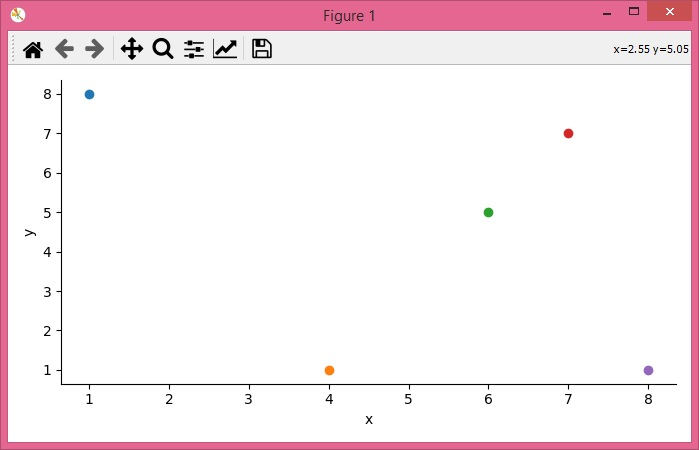# How to create multiple series scatter plots with connected points using seaborn?

To create multiple series scatter plots with connected points using seaborn, we can take the following steps −

## Steps

• Set the figure size and adjust the padding between and around the subplots.

• Create a Pandas data frame of two-dimensional, size-mutable, potentially heterogeneous tabular data.

• Multi-plot grid for plotting conditional relationships.

• Apply a plotting function to each facet's subset of the data.

• Plot the scatter and the data points with x and y data points.

• To display the figure, use show() method.

## Example

import pandas as pd
import seaborn as sns
from matplotlib import pyplot as plt

plt.rcParams["figure.figsize"] = [7.50, 3.50]
plt.rcParams["figure.autolayout"] = True

df = pd.DataFrame({"x": [4, 6, 7, 1, 8], "y": [1, 5, 7, 8, 1]})

g = sns.FacetGrid(df, hue="x", height=3.5)
g.map(plt.scatter, "x", "y")
g.map(plt.plot, "x", "y")

plt.show()

## Output

It will produce the following output −